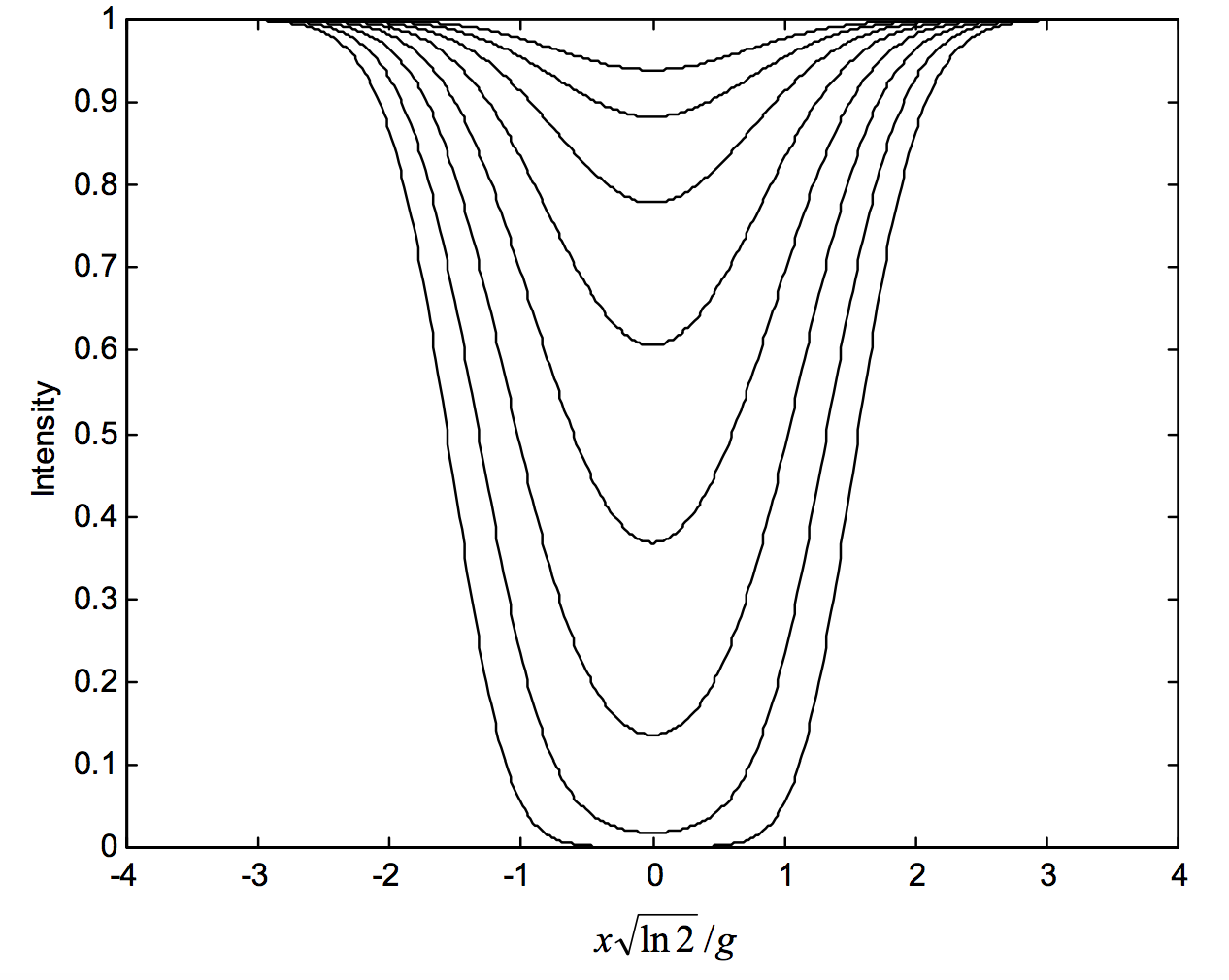# 11.4: Curve of Growth for Gaussian Profiles

$$\newcommand{\vecs}{\overset { \rightharpoonup} {\mathbf{#1}} }$$ $$\newcommand{\vecd}{\overset{-\!-\!\rightharpoonup}{\vphantom{a}\smash {#1}}}$$$$\newcommand{\id}{\mathrm{id}}$$ $$\newcommand{\Span}{\mathrm{span}}$$ $$\newcommand{\kernel}{\mathrm{null}\,}$$ $$\newcommand{\range}{\mathrm{range}\,}$$ $$\newcommand{\RealPart}{\mathrm{Re}}$$ $$\newcommand{\ImaginaryPart}{\mathrm{Im}}$$ $$\newcommand{\Argument}{\mathrm{Arg}}$$ $$\newcommand{\norm}{\| #1 \|}$$ $$\newcommand{\inner}{\langle #1, #2 \rangle}$$ $$\newcommand{\Span}{\mathrm{span}}$$ $$\newcommand{\id}{\mathrm{id}}$$ $$\newcommand{\Span}{\mathrm{span}}$$ $$\newcommand{\kernel}{\mathrm{null}\,}$$ $$\newcommand{\range}{\mathrm{range}\,}$$ $$\newcommand{\RealPart}{\mathrm{Re}}$$ $$\newcommand{\ImaginaryPart}{\mathrm{Im}}$$ $$\newcommand{\Argument}{\mathrm{Arg}}$$ $$\newcommand{\norm}{\| #1 \|}$$ $$\newcommand{\inner}{\langle #1, #2 \rangle}$$ $$\newcommand{\Span}{\mathrm{span}}$$$$\newcommand{\AA}{\unicode[.8,0]{x212B}}$$

By “gaussian profile” in the title of this section, I mean profiles that are gaussian in the optically-thin case; as soon as there are departures from optical thinness, there are also departures from the gaussian profile. Alternatively, the absorption coefficient and the optical depth are gaussian; the absorptance is not.

For an optically-thin thermally-broadened line, the optical thickness as a function of wavelength is given by

$\label{11.4.1}\tau (x)=\tau (0)\text{exp}\left ( -\frac{x^2\ln 2}{g^2}\right ) ,$

where the HWHM is

$\label{11.4.2}g=\frac{V_m \lambda_0 \sqrt{\ln 2}}{c}.$

Here $$V_\text{m}$$ is the modal speed. (See Chapter 10 for details.) The line profiles, as calculated from equations 11.3.4 and \ref{11.4.1}, are shown in figure XI.2 for the following values of the optical thickness at the line centre: $$\tau (0)=\frac{1}{16},\frac{1}{8},\frac{1}{4},\frac{1}{2},1,2,4,8.$$

On combining equations 11.3.4 and \ref{11.4.1} and \ref{11.4.2}, we obtain the following expression for the equivalent width:

$\label{11.4.3}W=2\int_0^\infty \left ( 1-\text{exp}\left \{-\tau (0)e^{-x^2\ln 2/g^2}\right \}\right )\,dx,$

or

$\label{11.4.4}W=\frac{2V_\text{m}\lambda_0}{c}\int_0^\infty \left ( 1-\text{exp}\left \{-\tau(0)e^{-\Delta^2}\right \}\right )\,d\Delta,$

where

$\label{11.4.5}\Delta =\frac{x\sqrt{\ln 2}}{g}=\frac{cx}{V_\text{m}\lambda_0}.$

The half-width at half maximum (HWHM) of the expression \ref{11.4.1} for the optical thickness corresponds to $$\Delta = \sqrt{\ln 2} = 0.8326$$. For the purposes of practical numerical integration of Equation \ref{11.4.4}, I shall integrate from $$\Delta = -5\text{ to }+5$$ that is to say from $$\pm 6$$ times the HWHm. One can appreciate from figure XI.2 that going outside these limits will not contribute significantly to the equivalent width. I shall calculate the equivalent width for central optical depths ranging from $$1/20 (\log \tau (0) = −1.3)\text{ to }10^5 (\log \tau (0) = 5.0)$$.

What we have in figure XI.3 is a curve of growth for thermally broadened lines (or lines that, in the optically thin limit, have a gaussian profile, which could include a thermal and a microturbulent component). I have plotted this from $$\log \tau(0) = -1.3\text{ to }+5.0$$; that is $$\tau(0) = 0.05\text{ to }10^5$$.$$\text{FIGURE XI.2}$$$$\text{FIGURE XI.3}$$

This page titled 11.4: Curve of Growth for Gaussian Profiles is shared under a CC BY-NC 4.0 license and was authored, remixed, and/or curated by Jeremy Tatum via source content that was edited to the style and standards of the LibreTexts platform; a detailed edit history is available upon request.